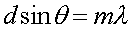Diffraction gratings

Diffraction gratings are glass plates with many parallel lines etched into the surface (or more cheaply, plastic copies of the same). Any adjacent pair of lines will act as a two slit setup, so the two slit forumlawill apply here as well to find the angles where a maximum occurs in the interference pattern. The difference is that the pattern made by the diffraction grating is much brighter and much sharper. To understand why the maxima are brighter, suppose that 100 slits are involved. The electric and magnetic fields at the maxima are then 100 times larger than they would be with just one slit (25 times larger than with 2 slits). Since the intensity is proportional to the square of E and/or B, the intensity is 10000 times larger than it would be with just 1 slit (2500 times larger than with 2 slits).

The maxima must be very sharp, because the total power going through 100 slits is only 100 times as large as the power going through 1 slit. So if the maxima are roughly 10000 times as bright, they must be roughly 1/100 times as broad, in view of the conservation of energy.

Interference and diffraction index      examples        Lecture index